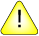This version of this document is no longer maintained. For the latest documentation, see http://www.qnx.com/developers/docs.

isnan(), isnanf()

Test for not-a-number (NAN)

Synopsis:

#include <math.h>

int isnan ( double x );

int isnanf ( float x );

Arguments:

x
The number you want to test.

Library:

libm

Use the -l m option to qcc to link against this library.

Description:

The isnan() and isnanf() functions determine if x is Not-A-Number (NAN).

Returns:

1
The value of x is NAN.
=/= 1
The value of x is a number.

Examples:

#include <stdio.h>
#include <stdlib.h>
#include <errno.h>
#include <inttypes.h>
#include <math.h>
#include <fpstatus.h>

int main(int argc, char** argv)
{
double a, b, c, d;

a = 2;
b = -0.5;
c = NAN;
d = 1.0/0.0;
printf("%f is %s \n", a,
(isnan(a)) ? "not a number" : "a number");
printf("%f is %s \n", b,
(isnan(b)) ? "not a number" : "a number");
printf("%f is %s \n", c,
(isnan(c)) ? "not a number" : "a number");
printf("%f is %s \n", d,
(isnan(d)) ? "not a number" : "a number");
return EXIT_SUCCESS;
}

produces the output:

2.000000 is a number
-0.500000 is a number
NAN is not a number
Inf is a number

Classification:

isnan() is POSIX 1003.1; isnanf() is Unix

Safety:
Cancellation point No
Interrupt handler No
Signal handler No